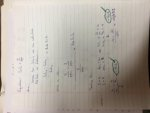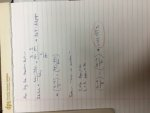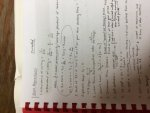MacroEcon-- Calculating Change in Stnd. of Living

Numberphile

New member
This is really a straight math question. Here are the equations I've been working with.

SoL = (Y/popn.) and SoL = (Y/L)*(L/popn.)

Where: Y--> output ; L--> # of working people

When given enough information to calculate percent change values for two of the variables, we use the principle shown below to solve:

%▲(x/y) = %▲x - %▲y

and

%▲(x*y) = %▲x + %▲y

For example:

%▲(SoL) = %▲(Y/L) + %▲(L/popn.)

I remember learning this this application in high school, but I'm highly unsatisfied with just applying it without knowing why this rule works. Why does this even work?

​Thanks to all in advance.

Attachments

• 6.2 KB Views: 1

Harry_the_cat

Senior Member
Just testing this "principle" out:

If old x = 10 and new x = 12, then %change in x is 20%.

If old y = 8 and new y = 10, then %change in y is 25%.

Old xy = 10*8 = 80 and new xy = 12 *10 =120 , then %change in xy is 50%.

But 20% + 25% does not equal 50%.

Am I misunderstanding something, or does the "principle" not work?

Numberphile

New member
I am also having the same issue... when I ran the numbers, the two equations didn't match results. Yet, my professor clearly has this written down for the test and I used it to find the solutions for homework (matched the answer key) earlier today...I apologize for the photos being sideways.

Dr.Peterson

Elite Member
When given enough information to calculate percent change values for two of the variables, we use the principle shown below to solve:

%▲(x/y) = %▲x - %▲y

and

%▲(x*y) = %▲x + %▲y

For example:

%▲(SoL) = %▲(Y/L) + %▲(L/popn.)

I remember learning this this application in high school, but I'm highly unsatisfied with just applying it without knowing why this rule works. Why does this even work?

This is an approximation that is appropriate for small changes. Similar facts are used in the study of "propagation of error" (but with different signs).

The percent change in x/y is $$\displaystyle \dfrac{\frac{x+\Delta x}{y + \Delta y}}{\frac{x}{y}}-1 = \dfrac{1+\frac{\Delta x}{x}}{1 + \frac{\Delta y}{y}}-1 = (1+\frac{\Delta x}{x})(1 - \frac{\Delta y}{y} + (\frac{\Delta y}{y})^2 - \dots)-1 \approx (1+\frac{\Delta x}{x})(1 - \frac{\Delta y}{y}) - 1 \approx \frac{\Delta x}{x} - \frac{\Delta y}{y}$$

For example, if x and y were 5 and 1 and increased by 3% to 5.15 and by 1% to 1.01, the percent change in x/y would be (5.15/1.01 - 5/1)/(5/1) - 1 = (5.0099 - 5)/5 = 0.0198 = 2%, which is 3% - 1%, approximately.

I would certainly hope that the word "approximate" was mentioned at some point!

Numberphile

New member
This is an approximation that is appropriate for small changes. Similar facts are used in the study of "propagation of error" (but with different signs).

The percent change in x/y is $$\displaystyle \dfrac{\frac{x+\Delta x}{y + \Delta y}}{\frac{x}{y}}-1 = \dfrac{1+\frac{\Delta x}{x}}{1 + \frac{\Delta y}{y}}-1 = (1+\frac{\Delta x}{x})(1 - \frac{\Delta y}{y} + (\frac{\Delta y}{y})^2 - \dots)-1 \approx (1+\frac{\Delta x}{x})(1 - \frac{\Delta y}{y}) - 1 \approx \frac{\Delta x}{x} - \frac{\Delta y}{y}$$

For example, if x and y were 5 and 1 and increased by 3% to 5.15 and by 1% to 1.01, the percent change in x/y would be (5.15/1.01 - 5/1)/(5/1) - 1 = (5.0099 - 5)/5 = 0.0198 = 2%, which is 3% - 1%, approximately.

I would certainly hope that the word "approximate" was mentioned at some point!
Thank you so much for this explanation! It makes sense now-- the homework problems were with small changes consistently. I was so confused on why I could get matching answers with both methods on the homework, but couldn't get the correct answers using that method with numbers I chose randomly. I'm so gratified.

AND...

The word approximate was certainly never used in class. Nothing of the sorts, actually... I sit right in the front row. I'm sure most students will just use the formula and get the answers on the test as the Prof. wants but it made no sense to me.

Thanks again!!Dr.Peterson

Elite Member
Thank you so much for this explanation! It makes sense now-- the homework problems were with small changes consistently. I was so confused on why I could get matching answers with both methods on the homework, but couldn't get the correct answers using that method with numbers I chose randomly. I'm so gratified.

AND...

The word approximate was certainly never used in class. Nothing of the sorts, actually... I sit right in the front row. I'm sure most students will just use the formula and get the answers on the test as the Prof. wants but it made no sense to me.

Thanks again!!I'm not really surprised; mathematicians like precision and reasons, while practical people like procedures and rules, and may not distinguish between exact and approximate.

Congratulations -- you're a mathematician!